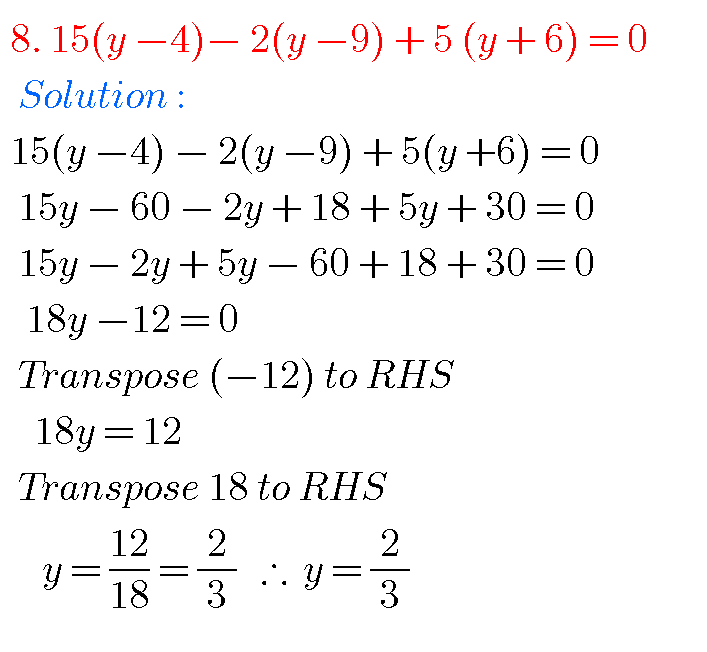## Ncert solutions for class 8 maths chapter 2,Linear Equations in One Variable solutions

NCERT SOLUTIONS FOR CLASS 8  MATHS CHAPTER 2 Class 8 Chapter 2 Linear Equations in One Variable Solutions Maths Ncert are given. First you study the textbook lesson very well. Observe and practice the example problems and solutions given in the textbook. LINEAR EQUATIONS IN ONE VARIABLE SOLUTIONS You can see the solutions of Chapter …